# OQPSK Modulator Baseband

Modulation using OQPSK method

• Library:
• Communications Toolbox / Modulation / Digital Baseband Modulation / PM

•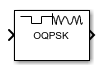## Description

The OQPSK Modulator Baseband block modulates the input signal using the offset quadrature phase shift keying (OQPSK) method and applies pulse shape filtering to the waveform. For more information, see Pulse Shaping Filter. The output is a baseband representation of the modulated signal.

For information about delays incurred by modulator-demodulator pair processing, see Modulation Delays.

## Ports

### Input

expand all

Input data, specified as an integer or bit column vector.

The input signal is processed based on the setting selected for Input type.

Data Types: `double`

### Output

expand all

Output baseband waveform, returned as a column vector of complex data.

## Parameters

expand all

### Modulation

Input type, specified as `Integer` or `Bit`.

• When you set Input type to `Integer`, the input can be a scalar value or column vector, the length of which is the number of input symbols.

• When you set Input type to `Bit`, the input width must be an integer multiple of two.

The output sample period is the period of each integer or bit pair in the input divided by Samples per symbol.

Phase offset from π/4, specified as a scalar in radians. The phase offset is applied to the zeroth point of the signal constellation before delay of quadrature component. After the OQPSK imaginary-component delay, the signal is normalized with unity power.

Example: Setting Phase offset (rad) to `pi/4` aligns the zeroth point of the QPSK signal constellation point on the axes, {(1,0), (0,j), (-1,0), (0,-j)}.

Signal constellation bit mapping, specified as `Gray`, `Binary`, or a custom 4-element numeric vector of integers with values from 0 to 3.

SettingConstellation Mapping for IntegersConstellation Mapping for BitsComment

`Gray`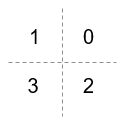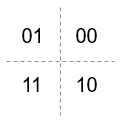The signal constellation mapping is Gray-encoded.

`Binary`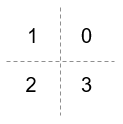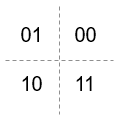The signal constellation mapping for the input integer `m` (0 ≤ `m` ≤ 3) is the complex value ```e(j*(PhaseOffset+π/4) + j*2*π*m/4)```.

Custom 4-element numeric vector of integers with values from 0 to 3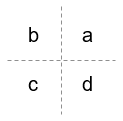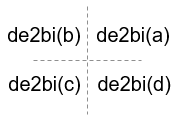Elements [a b c d] must be composed of the set of values [0, 1, 2, 3] in any order.

### Filtering

Select the filtering pulse shape: ```Half sine```, `Normal raised cosine`, `Root raised cosine`, or `Custom`.

Raised cosine filter rolloff factor, specified as a scalar in the range [`0`, `1`].

#### Dependencies

This property is enabled when Pulse shape is ```Normal raised cosine``` or ```Root raised cosine```.

Data Types: `double`

Filter length in symbols, specified as a scalar. An ideal raised cosine filter has an infinite impulse response. However, to realize a practical implementation of this filter, the object truncates the impulse response to Filter span (in symbols) symbols.

#### Dependencies

This property is enabled when Pulse shape is ```Normal raised cosine``` or ```Root raised cosine```.

Data Types: `double`

Filter numerator, specified as a row vector.

#### Dependencies

This parameter is enabled when Pulse shape is `Custom`.

Data Types: `double`

Number of samples per symbol, specified as a positive even integer.

Data Types: `double`

### Other Parameters

• `Enforce single-rate processing` — Executes the model, ensuring that the input and output signals have the same port sample time. The block implements the rate change by making a size change at the output when compared to the input. For integer inputs, the output width equals Samples per symbol times the number of symbols.

• `Allow multirate processing` — Executes the model, allowing the input and output signals to have different port sample times. The output sample time equals the symbol period divided by Samples per symbol.

Select the output data type: `double` or `single`.

## Block Characteristics

 Data Types `Boolean` | `double` | `integer` | `single` Multidimensional Signals `no` Variable-Size Signals `no`

expand all

## Extended Capabilities

### Topics

Introduced before R2006a